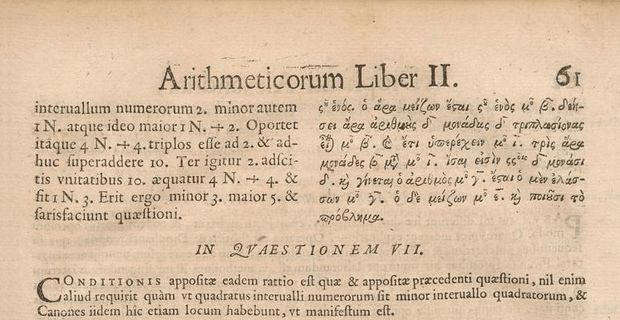# Fermat’s Last TheoremFermat’s last theorem was the last problem Pierre de Fermat ever claimed to have solved. Fermat often wrote in the margins of his books and one particular evening, while reading Arithmetica by the Greek mathematician Diophantus, he wrote “I have the answer to this equation, but the margin is too small to contain it”. Hours later that same night after writing this notation, Fermat died. Fermat was famous for writing in the margin of a books when he had figured out the correct answer for a problem, but for some reason he never provided the answer. Fermat was proven correct in each accounted notation by future mathematicians who came along posthumously. Fermat’s last claim however was unable to be solved and proven for centuries. Fermat’s last claim posited that the Pythagorean equation of a2 + b2 = c2 does not have an infinite number of solutions when the squared exponent of all 3 numbers is any number greater than 1 or 2 (e.g. it is impossible to separate a biquadrant or cube into 2 biquadrants or 2 cubes, only squared numbers can be separated into 2). Andew Wiles of Princeton University eventually solved this equation in 1993 but could not publish his work until 1995 due to an error. Wiles resolved this problem 3 centuries after Fermat as no other mathematician was able to figure it out